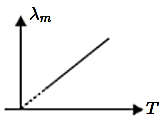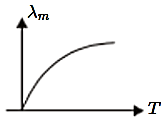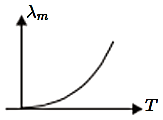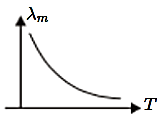One kilogram of ice at $0°$C is mixed with one kilogram of water at 80$°C$. The final temperature of the mixture is (Take: Specific heat of water = 4200 J $k{g}^{-1}{C}^{-1}$, Latent heat of ice = 336 kJ $k{g}^{-1}$)

(1) $0°$C              (2) $50°C$

(3) $40°C$           (4) $60°C$

Subtopic:  Calorimetry |
To view explanation, please take trial in the course below.
NEET 2022 - Target Batch - Aryan Raj Singh
To view explanation, please take trial in the course below.
NEET 2022 - Target Batch - Aryan Raj Singh

Difficulty Level:

The fastest mode of heat transfer is

1.  Conduction

3.  Convection

4.  all are  equally fast

To view explanation, please take trial in the course below.
NEET 2022 - Target Batch - Aryan Raj Singh
To view explanation, please take trial in the course below.
NEET 2022 - Target Batch - Aryan Raj Singh

Difficulty Level:

A temperature of 100 oF (Fahrenheit scale) is equal to T K (Kelvin scale). The value of T is:

1.  310.9

2.  37.8

3.  100

4.  122.4

Subtopic:  Temperature and Heat |
To view explanation, please take trial in the course below.
NEET 2022 - Target Batch - Aryan Raj Singh
To view explanation, please take trial in the course below.
NEET 2022 - Target Batch - Aryan Raj Singh

Difficulty Level:

A black body at temperature 300K radiates heat at the rate E. If its temperature is increased by 600K, the rate of radiation will increase to -

1.  16E

2.  64E

3.  81E

4.  256E

To view explanation, please take trial in the course below.
NEET 2022 - Target Batch - Aryan Raj Singh
To view explanation, please take trial in the course below.
NEET 2022 - Target Batch - Aryan Raj Singh

Difficulty Level:

A body cools down from  in 5 minutes. The temperature of the body will fall from  in a time:

1.  less than 5 minutes.

2.  equal to 5 minutes.

3.  more than 5 minutes.

4.  can't say anything like the temperature of surroundings is not known.

Subtopic:  Newton's Law of Cooling |
To view explanation, please take trial in the course below.
NEET 2022 - Target Batch - Aryan Raj Singh
To view explanation, please take trial in the course below.
NEET 2022 - Target Batch - Aryan Raj Singh

Difficulty Level:

If ${\lambda }_{m}$ is the wavelength, corresponding to which the radiant intensity of a block is at its maximum and its absolute temperature is T. Which of the following graph correctly represents the variation of T.

1.2.3.4.Subtopic:  Wien's Displacement Law |
To view explanation, please take trial in the course below.
NEET 2022 - Target Batch - Aryan Raj Singh
To view explanation, please take trial in the course below.
NEET 2022 - Target Batch - Aryan Raj Singh

Difficulty Level:

Heat capacity is equal to the product of:

1.  mass and gas constant

2.  mass and specific heat

3.  latent heat and volume of water

Subtopic:  Calorimetry |
To view explanation, please take trial in the course below.
NEET 2022 - Target Batch - Aryan Raj Singh
To view explanation, please take trial in the course below.
NEET 2022 - Target Batch - Aryan Raj Singh

Difficulty Level:

When a block of iron floats in Hg at $0°C$, a fraction ${K}_{1}$ of its volumen= is submerged, while at temperature of $60°C$ a fraction ${K}_{2}$ is seen to be submersed. If the coefficient of volume expansion of iron is ${\gamma }_{Fe}$ and that of mercury is ${\gamma }_{Hg}$, then the ratio $\frac{{K}_{1}}{{K}_{2}}$ can be expressed as:

1. $\frac{1+60{\gamma }_{Fe}}{1+60{\gamma }_{Hg}}$

2. $\frac{1-60{\gamma }_{Fe}}{1+60{\gamma }_{Hg}}$

3. $\frac{1+60{\gamma }_{Fe}}{1-60{\gamma }_{Hg}}$

4. $\frac{1+60{\gamma }_{He}}{1-60{\gamma }_{Fe}}$

Subtopic:  Thermal Expansion |
To view explanation, please take trial in the course below.
NEET 2022 - Target Batch - Aryan Raj Singh
To view explanation, please take trial in the course below.
NEET 2022 - Target Batch - Aryan Raj Singh

Difficulty Level:

Hot water cools from 60$°\mathrm{C}$ to 50$°\mathrm{C}$ in first 10 minutes and from 50$°\mathrm{C}$ to 42$°\mathrm{C}$ in next 10 minutes. The temperature of surrounding is :

1.

2.

3.

4.  $20°\mathrm{C}$

Subtopic:  Newton's Law of Cooling |
To view explanation, please take trial in the course below.
NEET 2022 - Target Batch - Aryan Raj Singh
To view explanation, please take trial in the course below.
NEET 2022 - Target Batch - Aryan Raj Singh

Difficulty Level:

A pendulum clock runs fast by 5 seconds per day at 20 oC and goes slow by 10 second per day at 35 oC. It shows the correct time at a temperature of:

1.  27.5 oC

2.  25 oC

3.  30 oC

4.  33 oC

Subtopic:  Thermal Expansion |
To view explanation, please take trial in the course below.
NEET 2022 - Target Batch - Aryan Raj Singh
To view explanation, please take trial in the course below.
NEET 2022 - Target Batch - Aryan Raj Singh

Difficulty Level: